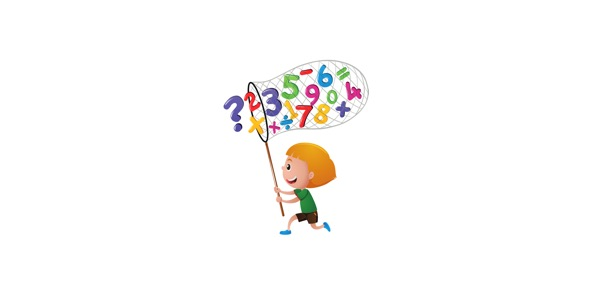# 6th Grade Math Mixed Review

13 Questions | Total Attempts: 59Settings• 1.
Write A short essay for what you have learned In this test And in this Lesson
• 2.
which is Equal?      6.5
• A.

Six and five tenth

• B.

Six point five

• 3.
Which is equal ?       0.3
• A.

Three tenths

• B.

Point three

• 4.
Which is equal ?        39.2
• A.

Thirty-nine point two

• B.

Thirty-nine and two tenths

• 5.
Which is equal ?     0.83
• A.

Eighty three

• B.

Point eighty - three

• C.

Eigthy-three hundredths

• 6.
Write the expanded form into standard form ?                                                                     [3•10]+ [9•1]+ [6•.1]
• 7.
86.582 _____  86.986
• A.

• B.

>

• C.

=

• 8.
36  ___  38
• A.

• B.

>

• C.

=

• 9.
36.5280000 ______=_______  36.528
• A.

True

• B.

False

• 10.
Decimals Is a Seperator ?
• A.

True

• B.

False

• 11.
Decimals are not a seporator for .3 ?
• A.

True

• B.

False

• 12.
Bouns #1     ( 5 points for correct answer )Which is a higher amount ?
• A.

36.39

• B.

36.39000

• C.

Same

• 13.
Bonus #2 ( 5 points for correct answer )Which Decimal Is Correct For two Hundred And sixty-nine Hundredths ?
• A.

200.69

• B.

200,69

• C.

200.069

Related TopicsBack to top